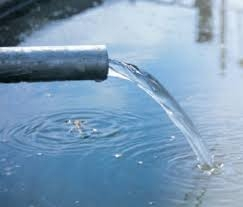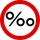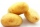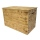# Metal tube

Calculate the metal tube mass 8dm long with the outer radius 5cm and the inner radius 4.5cm and 1cm3 of this metal is 9.5g.

Correct result:

m =  11.341 kg

#### Solution:

$r_{1}=4.5 \ \text{cm} \ \\ r_{2}=5 \ \text{cm} \ \\ l=8 \ dm \rightarrow cm=8 \cdot \ 10 \ cm=80 \ cm \ \\ \ \\ S=\pi \cdot \ r_{2}^2 - \pi \cdot \ r_{1}^2=3.1416 \cdot \ 5^2 - 3.1416 \cdot \ 4.5^2 \doteq 14.9226 \ \text{cm}^2 \ \\ \ \\ \ \\ V=S \cdot \ l=14.9226 \cdot \ 80 \doteq 1193.8052 \ \text{cm}^3 \ \\ \ \\ r=9.5 \ \text{g/cm}^3 \ \\ m_{1}=r \cdot \ V=9.5 \cdot \ 1193.8052 \doteq 11341.1495 \ \text{g} \ \\ \ \\ m=m_{1} \rightarrow kg=m_{1} / 1000 \ kg=11341.14947946 / 1000 \ kg=11.341 \ kg=11.341 \ \text{kg}$Our examples were largely sent or created by pupils and students themselves. Therefore, we would be pleased if you could send us any errors you found, spelling mistakes, or rephasing the example. Thank you!

Leave us a comment of this math problem and its solution (i.e. if it is still somewhat unclear...):Be the first to comment!Tips to related online calculators
Do you know the volume and unit volume, and want to convert volume units?
Tip: Our Density units converter will help you with the conversion of density units.
Do you want to convert mass units?

#### You need to know the following knowledge to solve this word math problem:

We encourage you to watch this tutorial video on this math problem:

## Next similar math problems:

• Copper Cu wireCopper wire with a diameter of 1 mm and a weight of 350 g is wound on a spool. Calculate its length if the copper density is p = 8.9 g/cm cubic.
• Three examples per-milleA. Calculate 1.5 ‰ from 4.5 liters of blood. Express the result in milliliters. B. Calculate three ‰ from € 50,000. C. The tray they brought breakfast to the landlord's bed is made of an alloy that contains 830 ‰ of silver. How much silver did they use
• Regular hexagonal prismCalculate the volume of a regular hexagonal prism whose body diagonals are 24cm and 25cm long.The surface of the regular quadrilateral prism is 8800 cm2, the base edge is 20 cm long. Calculate the volume of the prism
• Cuboid to cubeA cuboid with dimensions of 9 cm, 6 cm, and 4 cm has the same volume as a cube. Calculate the surface of this cube.
• Cuboid edgesCalculate the volume and surface of a cuboid whose edge lengths are in the ratio 2: 3: 4 and the longest edge measures 10cm.
• Cube surfce2volumeCalculate the volume of the cube if its surface is 150 cm2.
• Spherical segmentCalculate the volume of a spherical segment 18 cm high. The diameter of the lower base is 80 cm, the upper base 60 cm.
• The cubeThe cube has a surface area of 216 dm2. Calculate: a) the content of one wall, b) edge length, c) cube volume.
• The water tankThe water tank has the shape of a sphere with a radius of 2 m. How many liters of water will fit in the tank? How many kilograms of paint do we need to paint the tank, if we paint with 1 kg of paint 10 m2?
• Alcohol from potatoesIn the distillery, 10 hl of alcohol can make from 8 t of potatoes. The rectangular field with dimensions of 600 m and 200 m had a yield of 20 t of potatoes per hectare. How many square meters of area are potatoes grown to obtain one liter of alcohol?
• A bottleA bottle full of cola weighs 1,320 g. If we drink three-tenths of it, it will weigh 1,008g. How much does an empty bottle weigh?
• Concrete hatchThe concrete hatch for a round well has a diameter of 1300 mm and a thickness of 80 mm. Determine its weight in kg if the density of the concrete is 2545 kg/m3
• Wooden boxThe block-shaped box was placed on the ground, leaving a rectangular print with dimensions of 3 m and 2 m. When flipped over to another wall, a print with dimensions of 0.5 m and 3 m remained in the sand. What is the volume of the wooden box?
• Aquarium heightHow high does the water in the aquarium reach, if there are 36 liters of water in it? The length of the aquarium is 60 cm and the width is 4 dm.
• Surface and volumeFind the surface and volume of a cuboid whose dimensions are 1 m, 50 cm, and 6 dm.
• Water mixingWe have 520 ml of hot water and 640 ml of water at 48°C. What is the temperature of approximately hot water when the resulting mixture has a temperature of 65°C?